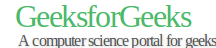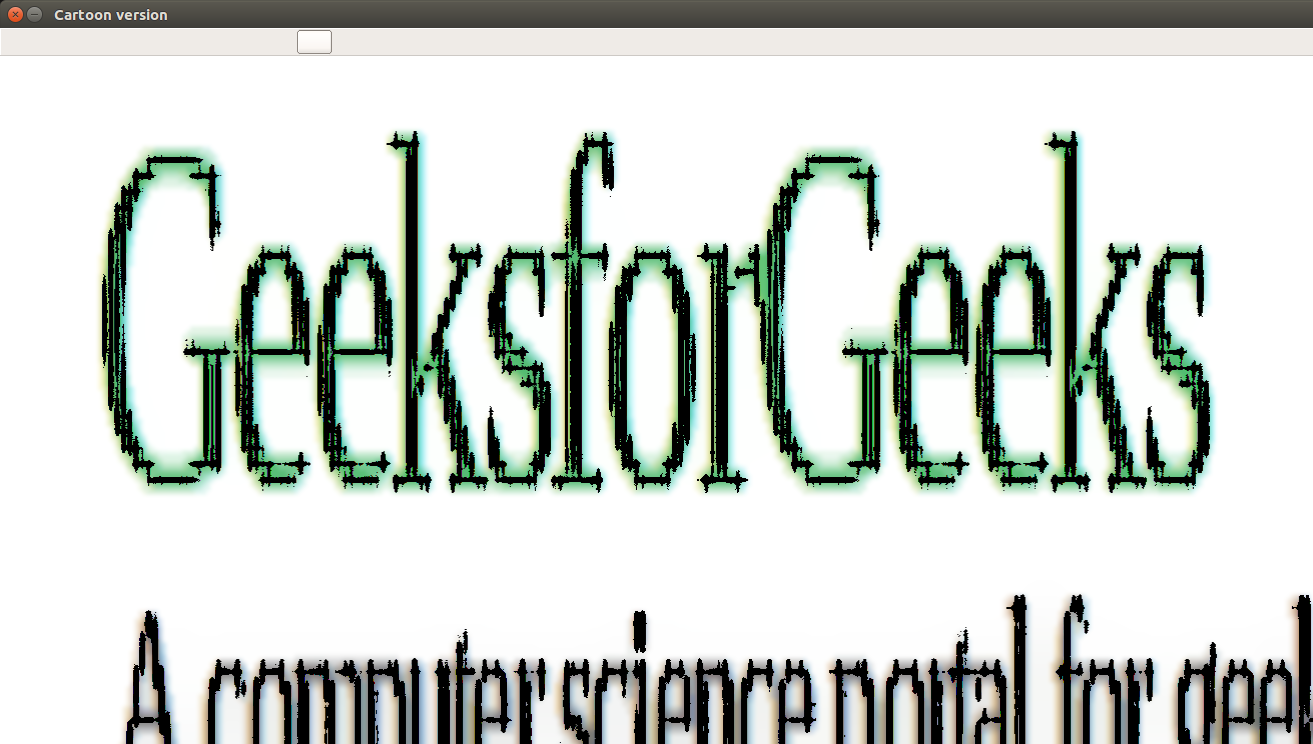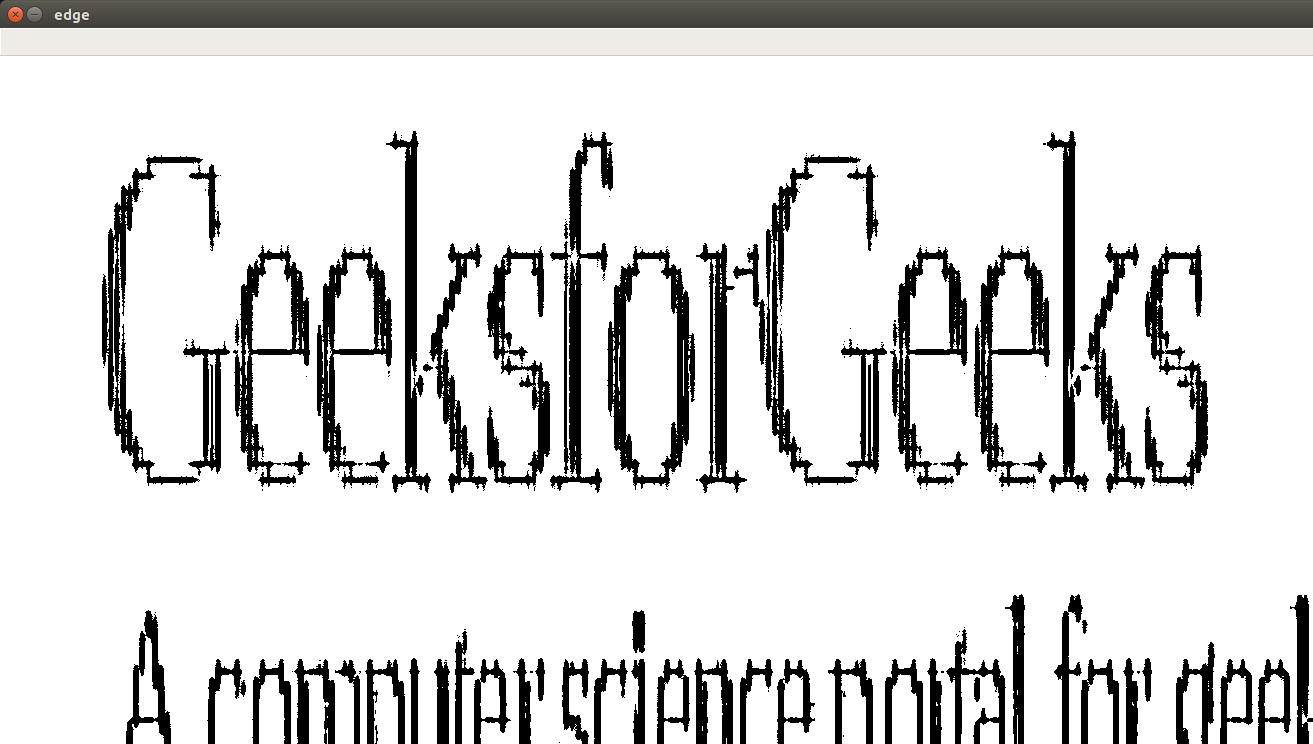Related Articles
Cartooning an Image using OpenCV – Python
• Difficulty Level : Hard
• Last Updated : 19 Nov, 2020

Computer Vision as you know (or even if you don’t) is a very powerful tool with immense possibilities. So, when I set up to prepare a comic of one of my friend’s college life, I soon realized that I needed something that would reduce my efforts of actually painting it but will retain the quality and I came up with the following solution.

Let’s see the result first –Original ImageCartooned VersionEdges obtained from the image (Adaptive Threshold result)

Let’s see the code:

 `class` `Cartoonizer: ``    ``"""Cartoonizer effect ``        ``A class that applies a cartoon effect to an image. ``        ``The class uses a bilateral filter and adaptive thresholding to create ``        ``a cartoon effect. ``    ``"""``    ``def` `__init__(``self``): ``        ``pass`` ` `    ``def` `render(``self``, img_rgb): ``        ``img_rgb ``=` `cv2.imread(img_rgb) ``        ``img_rgb ``=` `cv2.resize(img_rgb, (``1366``,``768``)) ``        ``numDownSamples ``=` `2`     `# number of downscaling steps ``        ``numBilateralFilters ``=` `50` `# number of bilateral filtering steps `` ` `        ``# -- STEP 1 -- `` ` `        ``# downsample image using Gaussian pyramid ``        ``img_color ``=` `img_rgb ``        ``for` `_ ``in` `range``(numDownSamples): ``            ``img_color ``=` `cv2.pyrDown(img_color) `` ` `        ``#cv2.imshow("downcolor",img_color) ``        ``#cv2.waitKey(0) ``        ``# repeatedly apply small bilateral filter instead of applying ``        ``# one large filter ``        ``for` `_ ``in` `range``(numBilateralFilters): ``            ``img_color ``=` `cv2.bilateralFilter(img_color, ``9``, ``9``, ``7``) `` ` `        ``#cv2.imshow("bilateral filter",img_color) ``        ``#cv2.waitKey(0) ``        ``# upsample image to original size ``        ``for` `_ ``in` `range``(numDownSamples): ``            ``img_color ``=` `cv2.pyrUp(img_color) ``        ``#cv2.imshow("upscaling",img_color) ``        ``#cv2.waitKey(0) `` ` `        ``# -- STEPS 2 and 3 -- ``        ``# convert to grayscale and apply median blur ``        ``img_gray ``=` `cv2.cvtColor(img_rgb, cv2.COLOR_RGB2GRAY) ``        ``img_blur ``=` `cv2.medianBlur(img_gray, ``3``) ``        ``#cv2.imshow("grayscale+median blur",img_color) ``        ``#cv2.waitKey(0) `` ` `        ``# -- STEP 4 -- ``        ``# detect and enhance edges ``        ``img_edge ``=` `cv2.adaptiveThreshold(img_blur, ``255``, ``                                        ``cv2.ADAPTIVE_THRESH_MEAN_C, ``                                        ``cv2.THRESH_BINARY, ``9``, ``2``) ``        ``#cv2.imshow("edge",img_edge) ``        ``#cv2.waitKey(0) `` ` `        ``# -- STEP 5 -- ``        ``# convert back to color so that it can be bit-ANDed with color image ``        ``(x,y,z) ``=` `img_color.shape ``        ``img_edge ``=` `cv2.resize(img_edge,(y,x)) ``        ``img_edge ``=` `cv2.cvtColor(img_edge, cv2.COLOR_GRAY2RGB) ``        ``cv2.imwrite(``"edge.png"``,img_edge) ``        ``#cv2.imshow("step 5", img_edge) ``        ``#cv2.waitKey(0) ``        ``#img_edge = cv2.resize(img_edge,(i for i in img_color.shape[:2])) ``        ``#print img_edge.shape, img_color.shape ``        ``return` `cv2.bitwise_and(img_color, img_edge) `` ` `tmp_canvas ``=` `Cartoonizer() `` ` `file_name ``=` `"Screenshot.png"` `#File_name will come here ``res ``=` `tmp_canvas.render(file_name) `` ` `cv2.imwrite(``"Cartoon version.jpg"``, res) ``cv2.imshow(``"Cartoon version"``, res) ``cv2.waitKey(``0``) ``cv2.destroyAllWindows() `

Explanation:

Basically, we are going to use a series of filters and image conversions.

• First we downscale the image and then apply bilateral filter to get a cartoon flavor. Then again we upscale the image.
• Next step is getting a blurred version of the original image. Now, we don’t want the colours to interfere in this process. We only want the blurring of the boundaries. For this, we first convert the image to gray – scale and then we apply the media blur filter.
• Next step is to identify the edges in the image and then add this to the previously modified images to get a sketch pen effect. For this first we are using adaptive threshold. You can experiment with other types of threshold techniques also. Because Computer Vision is all about experimenting. In step 5, we compile the final images obtained from the previous steps.

## Simpler Implementation –

 `# importing libraries``import` `cv2``import` `numpy as np`` ` `# reading image ``img ``=` `cv2.imread(``"koala.jpeg"``)``  ` `# Edges``gray ``=` `cv2.cvtColor(img, cv2.COLOR_BGR2GRAY)``gray ``=` `cv2.medianBlur(gray, ``5``)``edges ``=` `cv2.adaptiveThreshold(gray, ``255``, cv2.ADAPTIVE_THRESH_MEAN_C, ``                                         ``cv2.THRESH_BINARY, ``9``, ``9``)``  ` `# Cartoonization``color ``=` `cv2.bilateralFilter(img, ``9``, ``250``, ``250``)``cartoon ``=` `cv2.bitwise_and(color, color, mask``=``edges)``  ` `  ` `cv2.imshow(``"Image"``, img)``cv2.imshow(``"edges"``, edges)``cv2.imshow(``"Cartoon"``, cartoon)``cv2.waitKey(``0``)``cv2.destroyAllWindows()`

What you can do?
Experiment! Try changing the down sample steps, or the number of bilateral filters applied, or even the size of the filter, or the threshold technique to get the edges. Now, one thing to keep in mind. This process is a general one and will not give the best result for different images. That’s why, you should experiment with different values to get a feel of the whole process.

That’s all from my side! Auf Wiedersehen!

Vishwesh Shrimali is an Undergraduate Mechanical Engineering student at BITS Pilani. He fulfilsabout all the requirements not taught in his branch- white hat hacker, network security operator, and an ex – Competitive Programmer. As a firm believer in power of Python, his majority work has been in the same language. Whenever he get some time apart from programming, attending classes, watching CSI Cyber, he go for a long walk and play guitar in silence. His motto of life is – “Enjoy your life, ‘cause it’s worth enjoying!”